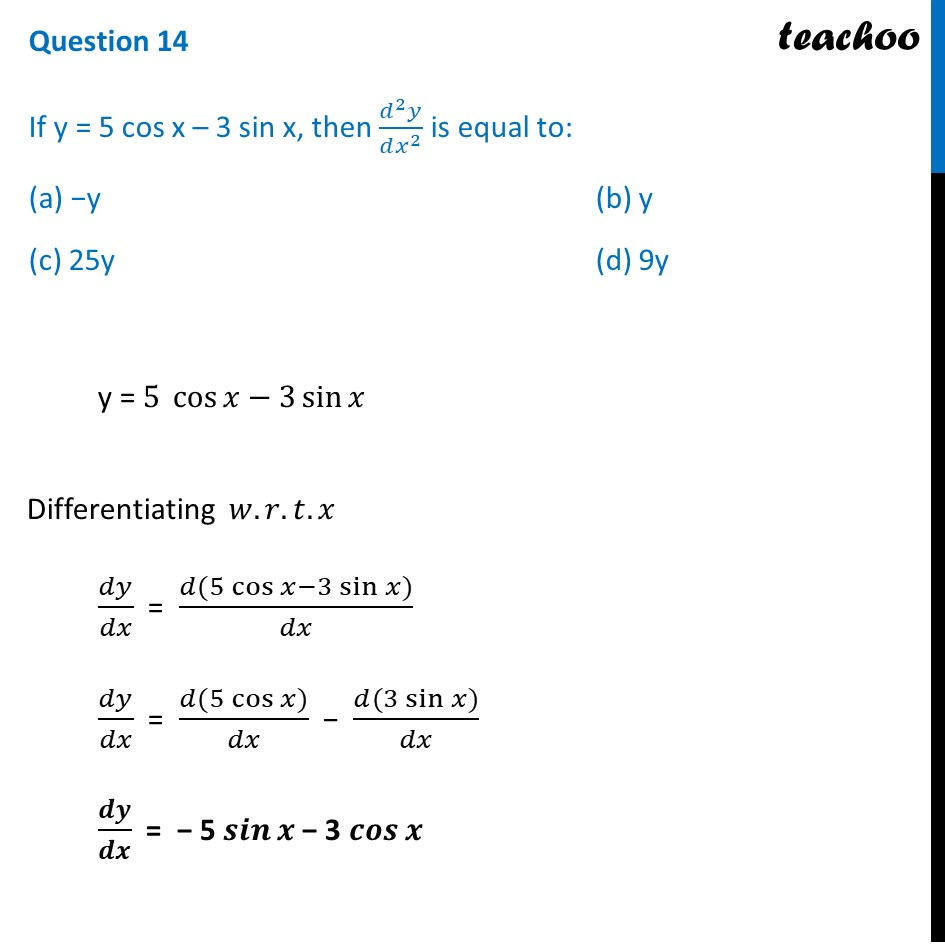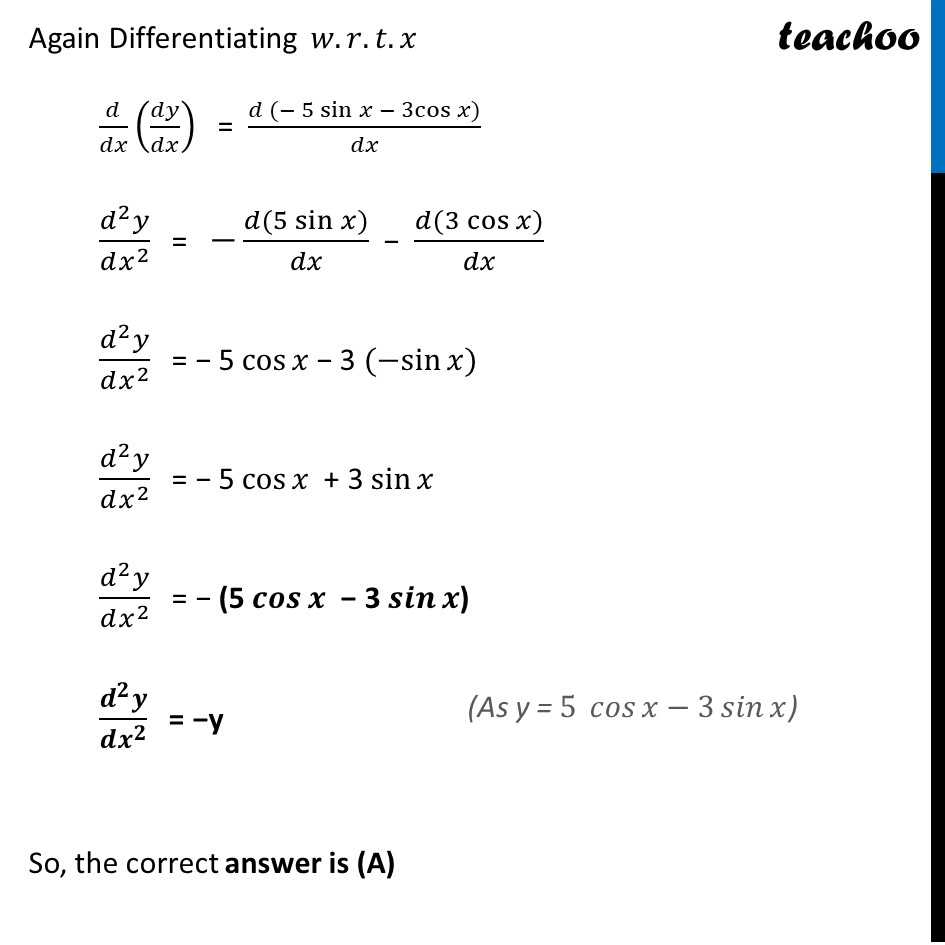CBSE Class 12 Sample Paper for 2022 Boards (MCQ Based - for Term 1)

Class 12
Solutions of Sample Papers and Past Year Papers - for Class 12 Boards

## (a) −y     (b) y (c) 25y   (d) 9y

This question is inspired from Ex 5.7, 11 - Chapter 5 Class 12 - Continuity and Differentiability### Transcript

Question 14 If y = 5 cos x – 3 sin x, then (𝑑^2 𝑦)/(𝑑𝑥^2 ) is equal to: (a) −y (b) y (c) 25y (d) 9y y = 5 cos⁡〖𝑥−3 sin⁡𝑥 〗 Differentiating 𝑤.𝑟.𝑡.𝑥 𝑑𝑦/𝑑𝑥 = (𝑑(5 cos⁡〖𝑥−3 sin⁡𝑥 〗))/𝑑𝑥 𝑑𝑦/𝑑𝑥 = (𝑑(5 cos⁡𝑥))/𝑑𝑥 − (𝑑(3 sin⁡𝑥))/𝑑𝑥 𝒅𝒚/𝒅𝒙 = − 5 𝒔𝒊𝒏⁡𝒙 − 3 𝒄𝒐𝒔⁡𝒙 Again Differentiating 𝑤.𝑟.𝑡.𝑥 𝑑/𝑑𝑥 (𝑑𝑦/𝑑𝑥) = (𝑑 〖(− 5 sin〗⁡𝑥 〖− 3cos〗⁡〖𝑥)〗)/𝑑𝑥 (𝑑^2 𝑦)/(𝑑𝑥^2 ) = −(𝑑(5 sin⁡𝑥))/𝑑𝑥 − (𝑑(3 cos⁡𝑥))/𝑑𝑥 (𝑑^2 𝑦)/(𝑑𝑥^2 ) = − 5 cos⁡𝑥 − 3 〖(−sin〗⁡〖𝑥)〗 (𝑑^2 𝑦)/(𝑑𝑥^2 ) = − 5 cos⁡𝑥 + 3 sin⁡𝑥 (𝑑^2 𝑦)/(𝑑𝑥^2 ) = − (5 𝒄𝒐𝒔⁡𝒙 − 3 𝒔𝒊𝒏⁡𝒙) (𝒅^𝟐 𝒚)/(𝒅𝒙^𝟐 ) = −y So, the correct answer is (A)